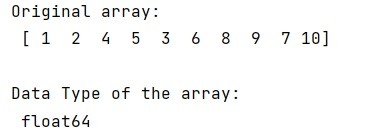# In-place type conversion of a NumPy array

Learn, how to implement in-place type conversion of a NumPy array?
Submitted by Pranit Sharma, on December 28, 2022

NumPy is an abbreviated form of Numerical Python. It is used for different types of scientific operations in python. Numpy is a vast library in python which is used for almost every kind of scientific or mathematical operation. It is itself an array which is a collection of various methods and functions for processing the arrays.

## NumPy Array - In-place Type Conversion

Whenever we apply a function to any array, we sometimes pass a parameter called inplace=True. The question is what is the significance of this parameter?

Whenever we make some changes to an array either index-wise or axis-wise (in the case of the multi-dimensional array), we either use the method in which we change the original value and return the changed value or we use a method where we do not change the entire data set and do the specific changes wherever required.

inplace=True means the data will be modified without returning a copy of the data or the original data.

Syntax:

```dataframe.some_operation(inplace=True)
```

But if inplace=False is passed then it will modify the value and also returns a copy of the data.

Syntax:

```dataframe.some_operation(inplace=False)
```

Here, we will use the astype() method to convert the data type where we can pass the argument copy=False.

Let us understand with the help of an example,

## Python code to demonstrate in-place type conversion of a NumPy array

```# Import numpy
import numpy as np

# Creating a numpy arrays
arr = np.array([1,2,4,5,3,6,8,9,7,10])

# Display original array
print("Original array:\n",arr,"\n")

# Convert the data type of the array
arr = arr.astype('float',copy=False)

# Display result
print("Data Type of the array:\n",arr.dtype)
```

Output: